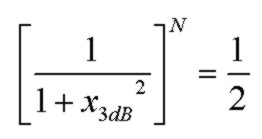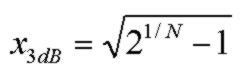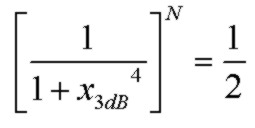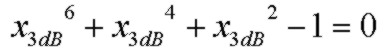Jan 24, 2013

This note provides expressions for the overall f3db frequency f3db_net for multi-stage unity-gain low-pass (LP) cascaded filters. N is the number of stages and each stage individually has the SAME -3db frequency f3db. The stages are assumed non-interacting. The overall f3db frequency is the half-power point of the combined filter stages. Results are given for first order filters (e.g. single pole RC filter) and 2nd order filters with the maximally-flat Butterworth response (Q = 1/√2).

x3db is the ratio f3db_net/f3db.
f3db is the -3db frequency of each individual stage.
N is the number of identical stages.

## First Order Single-Pole LP Filters

For N stages, the half-power point is given by:Some examples are:
```    N     x3db
1     1.0
2     0.6436
3     0.5098
```

## 2nd Order Double-Pole LP Filters with Q=1/√2

For N stages, the half-power point is given by:Some examples are:
```    N     x3db
1     1.0
2     0.8022
3     0.7140
```

(Note that the results for the 2nd order double-pole case are exactly the square-root of the results for the single-pole case)

## First Order and Second Order Filters

For the simple case of a single first-order filter cascaded with a single 2nd order filter (with Q=1/√2) with the same individual f3db frequency, the half-power point is given by:`     x3db = 0.73735 `